# FEM-Parameterized Synchronous Machine

Synchronous machine defined in terms of magnetic flux linkage

Since R2023a

•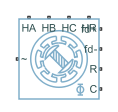Libraries:
Simscape / Electrical / Electromechanical / Synchronous

## Description

The FEM-Parameterized Synchronous Machine block implements the electrical and mechanical characteristics of an externally excited synchronous machine or hybrid excitation permanent magnet synchronous machine (PMSM) for which the magnetic flux linkage depends nonlinearly on the stator currents, field current, and rotor angle.

Use this block to represent an externally excited electric motor or machine that has a rotor field winding. This block captures detailed behaviour like saturation effects and cogging torques by importing finite-element-derived lookup tables from a motor design tool. You can also represent a hybrid excitation machine in which the permanent magnets and the field winding generate the rotor flux.

The FEM-Parameterized Synchronous Machine block uses flux linkage that you must calculate by using an external finite element (FE) tool. The block uses the A-phase flux linkage, which is a four-dimensional table that depends on the stator current magnitude, stator current phase advance, field winding current, and rotor angle. The block derives the field winding flux linkage by calculating the D-axis flux linkage from the A-phase, B-phase, and C-phase flux linkages and by subtracting the flux linkage when the field current is zero.

### Equations

This equation defines the back electromotive force (EMF) of the stator:

`$\left[\begin{array}{c}{e}_{A}\\ {e}_{B}\\ {e}_{C}\end{array}\right]=\left[\begin{array}{c}\frac{d{\Psi }_{A}}{dt}\\ \frac{d{\Psi }_{B}}{dt}\\ \frac{d{\Psi }_{C}}{dt}\end{array}\right]+{P}^{-1}\left[\begin{array}{c}0\\ 0\\ {L}_{0}\end{array}\right]\frac{d{i}_{0}}{dt},$`

where

• eA, eB, and eC are the stator winding voltages.

• ΨA, ΨB, and ΨC are the magnetic fluxes that link each stator winding.

• L0 is the value of the Stator zero-sequence inductance, L0 parameter.

• i0 is the zero-sequence current.

• P is the Park transform.

To keep the table dimension small, this block does not tabulate the stator flux linkages with the zero-sequence current. The block then adds the zero-sequence dynamics with a separate term. If you set the Winding type parameter to `Wye-wound` and do not enable the Expose neutral port parameter, then the zero-sequence dynamics are zero.

This equation defines the back EMF for the field winding of the rotor:

`${e}_{f}=\frac{d{\Psi }_{f}}{dt},$`

where:

• Ψf is the field winding flux linkage. These equations define the field winding flux linkage:

`$\begin{array}{c}\left[\begin{array}{c}{\Psi }_{d}\\ {\Psi }_{q}\\ {\Psi }_{0}\end{array}\right]=P\left(\theta \right)\left[\begin{array}{c}{\Psi }_{A}\left(I,B,\theta ,{i}_{f}\right)\\ {\Psi }_{B}\left(I,B,\theta ,{i}_{f}\right)\\ {\Psi }_{C}\left(I,B,\theta ,{i}_{f}\right)\end{array}\right]\\ {\Psi }_{f}\left(I,B,\theta ,{i}_{f}\right)={\Psi }_{d}\left(I,B,\theta ,{i}_{f}\right)-{\Psi }_{d}\left(I,B,\theta ,0\right)\end{array}$`

• ef is the field winding voltage.

• Ψd, Ψq, and Ψ0 are the d-axis, q-axis, and zero-sequence fluxes, respectively.

• ΨA(I,B,θ,if), ΨB(I,B,θ,if), and ΨC(I,B,θ,if) are the A-phase, B-phase, and C-phase flux linkages, respectively, tabulated with respect to I, B, θ, and if.

• I is the stator peak current magnitude.

• B is the stator current advance angle.

• θ is the rotor angle.

• Ψf (I,B,θ,if) is the field winding flux linkage tabulated with respect to I, B, θ, and if.

P is the Park transform, which depends on the option you choose for the Park’s convention for tabulated data parameter.

Note

The Park transform option of the Park’s convention for tabulated data parameter must match the version of the Park transform that you use in the FE tool to generate the flux linkage data.

Option 1: ```Q leads D, rotor angle measured from A-phase to D-axis```

Simscape™ Electrical™ motor and machine blocks use this convention internally and convert all other options to this format.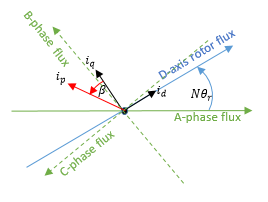• N is the value of the Number of pole pairs parameter.

• θr is the rotor angle.

• id and iq are the D-axis and Q-axis currents, respectively.

• ip is the current magnitude and is equal to $\sqrt{{i}_{d}^{2}+{i}_{q}^{2}}$.

• β is the current advance angle and is equal to ${\mathrm{tan}}^{-1}\left(-{i}_{d}/{i}_{q}\right)$.

The corresponding Park transform is

`$\left[\begin{array}{c}{i}_{d}\\ {i}_{q}\\ {i}_{0}\end{array}\right]=\frac{2}{3}\left[\begin{array}{ccc}\mathrm{cos}\left(N{\theta }_{r}\right)& \mathrm{cos}\left(N{\theta }_{r}-\frac{2\pi }{3}\right)& \mathrm{cos}\left(N{\theta }_{r}+\frac{2\pi }{3}\right)\\ -\mathrm{sin}\left(N{\theta }_{r}\right)& -\mathrm{sin}\left(N{\theta }_{r}-\frac{2\pi }{3}\right)& -\mathrm{sin}\left(N{\theta }_{r}+\frac{2\pi }{3}\right)\\ \frac{1}{2}& \frac{1}{2}& \frac{1}{2}\end{array}\right]\left[\begin{array}{c}{i}_{a}\\ \begin{array}{l}{i}_{b}\\ {i}_{c}\end{array}\end{array}\right]$`

where ia, ib, and ic are the A-phase, B-phase, and C-phase currents, respectively.

Option 2: ```Q leads D, rotor angle measured from A-phase to Q-axis```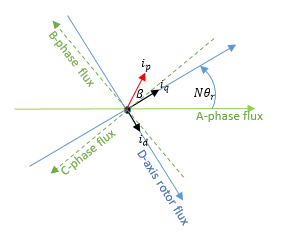• N is the value of the Number of pole pairs parameter.

• θr is the rotor angle.

• id and iq are the D-axis and Q-axis currents, respectively.

• ip is the current magnitude and is equal to $\sqrt{{i}_{d}^{2}+{i}_{q}^{2}}$.

• β is the current advance angle and is equal to ${\mathrm{tan}}^{-1}\left(-{i}_{d}/{i}_{q}\right)$.

The corresponding Park transform is

`$\left[\begin{array}{c}{i}_{d}\\ {i}_{q}\\ {i}_{0}\end{array}\right]=\frac{2}{3}\left[\begin{array}{ccc}\mathrm{sin}\left(N{\theta }_{r}\right)& \mathrm{sin}\left(N{\theta }_{r}-\frac{2\pi }{3}\right)& \mathrm{sin}\left(N{\theta }_{r}+\frac{2\pi }{3}\right)\\ \mathrm{cos}\left(N{\theta }_{r}\right)& \mathrm{cos}\left(N{\theta }_{r}-\frac{2\pi }{3}\right)& \mathrm{cos}\left(N{\theta }_{r}+\frac{2\pi }{3}\right)\\ \frac{1}{2}& \frac{1}{2}& \frac{1}{2}\end{array}\right]\left[\begin{array}{c}{i}_{a}\\ \begin{array}{l}{i}_{b}\\ {i}_{c}\end{array}\end{array}\right]$`

where ia, ib, and ic are the A-phase, B-phase, and C-phase currents, respectively.

Option 3: ```D leads Q, rotor angle measured from A-phase to D-axis```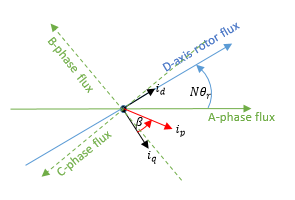• N is the value of the Number of pole pairs parameter.

• θr is the rotor angle.

• id and iq are the D-axis and Q-axis currents, respectively.

• ip is the current magnitude and is equal to $\sqrt{{i}_{d}^{2}+{i}_{q}^{2}}$.

• β is the current advance angle and is equal to ${\mathrm{tan}}^{-1}\left(-{i}_{d}/{i}_{q}\right)$.

The corresponding Park transform is

`$\left[\begin{array}{c}{i}_{d}\\ {i}_{q}\\ {i}_{0}\end{array}\right]=\frac{2}{3}\left[\begin{array}{ccc}\mathrm{cos}\left(N{\theta }_{r}\right)& \mathrm{cos}\left(N{\theta }_{r}-\frac{2\pi }{3}\right)& \mathrm{cos}\left(N{\theta }_{r}+\frac{2\pi }{3}\right)\\ \mathrm{sin}\left(N{\theta }_{r}\right)& \mathrm{sin}\left(N{\theta }_{r}-\frac{2\pi }{3}\right)& \mathrm{sin}\left(N{\theta }_{r}+\frac{2\pi }{3}\right)\\ \frac{1}{2}& \frac{1}{2}& \frac{1}{2}\end{array}\right]\left[\begin{array}{c}{i}_{a}\\ \begin{array}{l}{i}_{b}\\ {i}_{c}\end{array}\end{array}\right]$`

where ia, ib, and ic are the A-phase, B-phase, and C-phase currents, respectively.

Option 4: ```D leads Q, rotor angle measured from A-phase to Q-axis```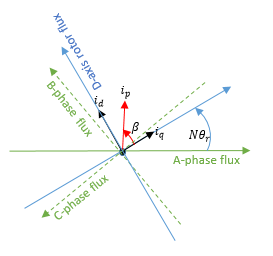• N is the value of the Number of pole pairs parameter.

• θr is the rotor angle.

• id and iq are the D-axis and Q-axis currents, respectively.

• ip is the current magnitude and is equal to $\sqrt{{i}_{d}^{2}+{i}_{q}^{2}}$.

• β is the current advance angle and is equal to ${\mathrm{tan}}^{-1}\left(-{i}_{d}/{i}_{q}\right)$.

The corresponding Park transform is

`$\left[\begin{array}{c}{i}_{d}\\ {i}_{q}\\ {i}_{0}\end{array}\right]=\frac{2}{3}\left[\begin{array}{ccc}-\mathrm{sin}\left(N{\theta }_{r}\right)& -\mathrm{sin}\left(N{\theta }_{r}-\frac{2\pi }{3}\right)& -\mathrm{sin}\left(N{\theta }_{r}+\frac{2\pi }{3}\right)\\ \mathrm{cos}\left(N{\theta }_{r}\right)& \mathrm{cos}\left(N{\theta }_{r}-\frac{2\pi }{3}\right)& \mathrm{cos}\left(N{\theta }_{r}+\frac{2\pi }{3}\right)\\ \frac{1}{2}& \frac{1}{2}& \frac{1}{2}\end{array}\right]\left[\begin{array}{c}{i}_{a}\\ \begin{array}{l}{i}_{b}\\ {i}_{c}\end{array}\end{array}\right]$`

where ia, ib, and ic are the A-phase, B-phase, and C-phase currents, respectively.

### Calculating Iron Losses

The FEM-Parameterized Synchronous Machine block models iron losses by using the Steinmetz equation. The Steinmetz equation scales for different motor speeds or electrical frequencies, so the block requires iron loss data only as a function of motor currents.

The block tabulates the iron losses coefficients with the Peak current magnitude vector, I, Current advance angle vector, B, and Field current vector, If parameters:

`$\begin{array}{l}{P}_{rotor}\left(f\right)={k}_{hr}\left({I}_{p},\beta ,If\right)f+{k}_{Jr}\left({I}_{p},\beta ,If\right){f}^{2}+{k}_{er}\left({I}_{p},\beta ,If\right){f}^{1.5}\\ {P}_{stator}\left(f\right)={k}_{hs}\left({I}_{p},\beta ,If\right)f+{k}_{Js}\left({I}_{p},\beta ,If\right){f}^{2}+{k}_{es}\left({I}_{p},\beta ,If\right){f}^{1.5}\end{array}$`

where:

• f is the electrical frequency, in Hz.

• khr(Ip,β,If) is the Rotor hysteresis loss coefficient, k_hr(I,B,If) parameter value.

• kJr(Ip,β,If) is the Rotor eddy current loss coefficient, k_Jr(I,B,If) parameter value.

• ker(Ip,β,If) is the Rotor excess current loss coefficient, k_er(I,B,If) parameter value.

• khs(Ip,β,If) is the Stator hysteresis loss coefficient, k_hs(I,B,If) parameter value.

• kJs(Ip,β,If) is the Stator eddy current loss coefficient, k_Js(I,B,If) parameter value.

• kes(Ip,β,If) is the Stator excess current loss coefficient, k_es(I,B,If) parameter value.

### Modeling Thermal Effects

You can expose thermal ports to model the effects of losses that convert power to heat. To expose the thermal ports, in the Thermal Ports section, enable the Model thermal effects parameter.

For more information about using thermal ports in actuator blocks, see Simulating Thermal Effects in Rotational and Translational Actuators.

### Variables

To set the priority and initial target values for the block variables before simulation, use the Initial Targets section in the block dialog box or Property Inspector. For more information, see Set Priority and Initial Target for Block Variables.

Nominal values provide a way to specify the expected magnitude of a variable in a model. Using system scaling based on nominal values increases the simulation robustness. You can specify nominal values using different sources, including the Nominal Values section in the block dialog box or Property Inspector. For more information, see System Scaling by Nominal Values.

## Assumptions and Limitations

If you model a hybrid-excitation machine, the block does not modulate the permanent magnet flux linkage with temperature.

## Ports

### Conserving

expand all

Expandable three-phase port associated with the stator windings. For more information, see Three-Phase Ports.

#### Dependencies

To enable this port, set Electrical connection to ```Composite three-phase ports```.

Electrical conserving port associated with the A phase.

#### Dependencies

To enable this port, set Electrical connection to ```Expanded three-phase ports```.

Electrical conserving port associated with the B phase.

#### Dependencies

To enable this port, set Electrical connection to ```Expanded three-phase ports```.

Electrical conserving port associated with the C phase.

#### Dependencies

To enable this port, set Electrical connection to ```Expanded three-phase ports```.

Electrical conserving port associated with the neutral phase.

#### Dependencies

To enable this port, enable the Expose neutral port parameter.

Electrical conserving port associated with the field winding positive terminal.

Electrical conserving port associated with the field winding negative terminal.

Mechanical rotational conserving port associated with the machine rotor.

Mechanical rotational conserving port associated with the machine case.

Thermal conserving port associated with winding A.

#### Dependencies

To enable this port, in the Thermal Ports section, enable the Model thermal effects parameter.

Thermal conserving port associated with winding B.

#### Dependencies

To enable this port, in the Thermal Ports section, enable the Model thermal effects parameter.

Thermal conserving port associated with winding C.

#### Dependencies

To enable this port, in the Thermal Ports section, enable the Model thermal effects parameter.

Thermal conserving port associated with the rotor.

#### Dependencies

To enable this port, in the Thermal Ports section, enable the Model thermal effects parameter.

## Parameters

expand all

### Flux & Torque

Option to specify composite or expanded three-phase ports.

Configuration of the stator windings, specified as one of these values:

• `Wye-wound` — Specify wye-wound stator windings.

• `Delta-wound` — Specify delta-wound stator windings. The A-phase is connected between ports a and b, the B-phase between ports b and c, and the C-phase between ports c and a.

Number of machine pole pairs.

Order and reference angle for the Park transform that the block uses to map the dq data to the three windings.

• ```Q leads D, rotor angle measured from A-phase to D-axis``` — Apply a quadrature-direct transformation and measure the angle with respect to the D axis.

• ```Q leads D, rotor angle measured from A-phase to Q-axis``` — Apply a quadrature-direct transformation and measure the angle with respect to the Q axis.

• ```D leads Q, rotor angle measured from A-phase to D-axis``` — Apply a direct-quadrature transformation and measure the angle with respect to the D axis.

• ```D leads Q, rotor angle measured from A-phase to Q-axis``` — Apply a direct-quadrature transformation and measure the angle with respect to the Q axis.

Row vector of current magnitudes at which the block tabulates the flux linkage. The first element must be `0`. The second current value must be small relative to the current values at which magnetic saturation begins to occur. This restriction is necessary because the derived flux partial derivatives are ill-defined at zero current, and the block calculates them at this first nonzero current instead.

Row vector of current advance angle values at which the block calculates the flux linkage. The current advance angle is the angle by which the current leads the Q-axis.

Row vector of field current values at which the block calculates the flux linkage. The first element must be `0`.

Vector of rotor angles at which the block tabulates the flux linkage. The first element of this vector must be `0`. The number of pole pairs is 360/Θmax, where Θmax is expressed in degrees. The default value corresponds to a four pole-pair motor.

4-D array of A-phase flux linkage values as a function of the Peak current magnitude vector, I, Current advance angle vector, B, Field current vector, If, and Rotor angle vector, theta parameters.

If your flux data is in a different order, use the `permute` function to reorder it. For an example of this reordering, see the `ee_ece_table ` function in Import IPMSM Flux Linkage Data from ANSYS Maxwell.

4-D array of the electromagnetic torque applied to the rotor as a function of the Peak current magnitude vector, I, Current advance angle vector, B, Field current vector, If, and Rotor angle vector, theta.

If your flux data is in a different order,use the `permute` function to reorder it. For an example of this reordering, see the `ee_ece_table ` function in Import IPMSM Flux Linkage Data from ANSYS Maxwell.

Interpolation method for approximating the output value when the input value is between two consecutive grid points.

• `Linear` — Use an extension of the linear algorithm for multidimensional interpolation. Select this option to get the best performance.

• `Smooth` — Use a modified Akima interpolation algorithm. Select this option to produce a continuous surface with continuous first-order derivatives.

For more information on interpolation methods, see the PS Lookup Table (4D) block reference page.

Option to expose the neutral port of the block.

If you expose the neutral port, the block also models the zero-sequence currents and parameterizes the zero sequence by using the Stator zero-sequence inductance, L0 parameter.

#### Dependencies

To enable this parameter, set Winding type to `Wye-wound`.

Zero-sequence inductance.

#### Dependencies

To enable this parameter, set Winding type to `Delta-Wound` or enable the Expose neutral port parameter.

### Resistance

Resistance of each phase of the stator windings.

Resistance of the field winding.

Coefficient α in the equation relating resistance to temperature for all three windings. For more information, see Thermal Model for Actuator Blocks. The default value is for copper.

#### Dependencies

To enable this parameter, in the Thermal Ports section, enable the Model thermal effects parameter.

Temperature at which the block measures the field winding and stator resistances.

#### Dependencies

To enable this parameter, in the Thermal Ports section, enable the Model thermal effects parameter.

### Iron Losses

Hysteresis loss coefficient of the rotor. The value depends on the current magnitude, current phase advance, and field current. The block uses this value in the Steinmetz equation.

Eddy current loss coefficient of the rotor. The value depends on the current magnitude, current phase advance, and field current. The block uses this value in the Steinmetz equation.

Excess current loss coefficient of the rotor. The value depends on the current magnitude, current phase advance, and field current. The block uses this value in the Steinmetz equation.

Hysteresis loss coefficient of the stator. The value depends on the current magnitude, current phase advance, and field current. The block uses this value in the Steinmetz equation.

Eddy current loss coefficient of the stator. The value depends on the current magnitude, current phase advance, and field current. The block uses this value in the Steinmetz equation.

Excess current loss coefficient of the stator. The value depends on the current magnitude, current phase advance, and field current. The block uses this value in the Steinmetz equation.

### Mechanical

Inertia of the rotor attached to mechanical translational port R.

Rotary damping.

### Thermal Ports

Option to expose the thermal ports and model the effects of losses that convert power to heat.

Thermal mass value for each stator winding. The thermal mass is the energy required to raise the temperature by one degree.

Thermal mass of the rotor. The thermal mass is the energy required to raise the temperature of the rotor by one degree.

## Version History

Introduced in R2023a